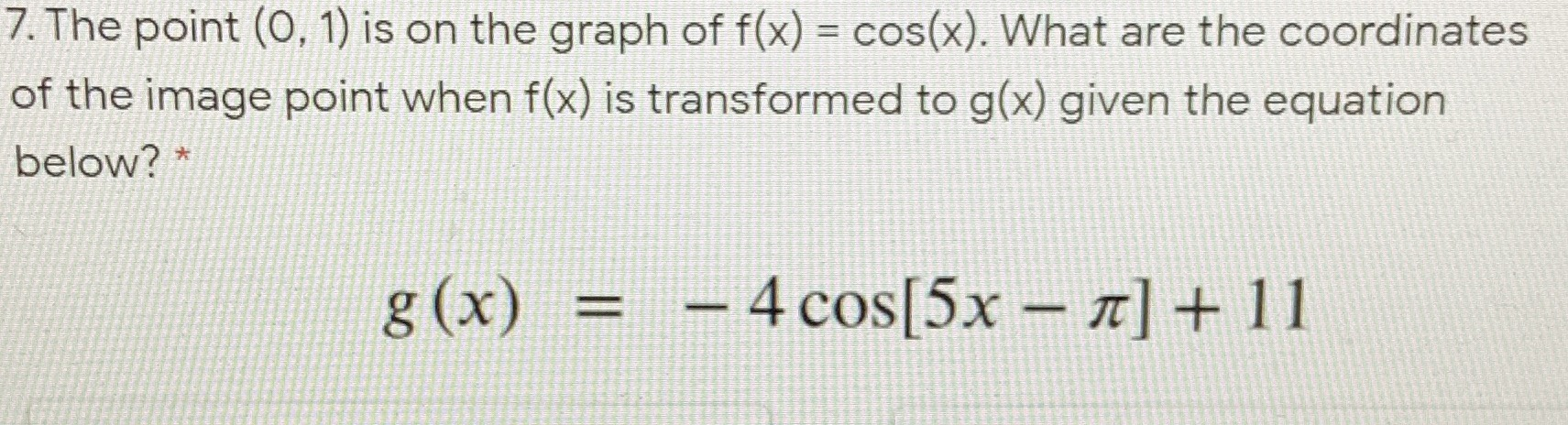### ¿Todavía tienes preguntas de matemáticas?

Pregunte a nuestros tutores expertos
Algebra
Pregunta7. The point $$( 0,1 )$$ is on the graph of $$f ( x ) = \cos ( x )$$ . What are the coordinates of the image point when $$f ( x )$$ is transformed to $$g ( x )$$ given the equation below? *

$$g ( x ) = - 4 \cos [ 5 x - \pi ] + 11$$